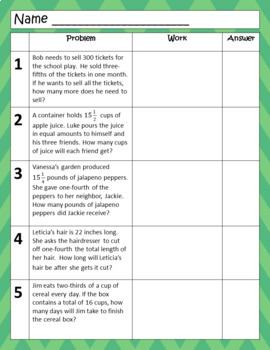DID YOU KNOW:
Seamlessly assign resources as digital activities

Learn how in 5 minutes with a tutorial resource. Try it Now

Learn More# Multiplying and Dividing Fractions Word Problems Scrambled Answers ActivityGrade Levels
5th - 7th, Homeschool
Subjects
Standards
Resource Type
Formats Included
• PDF
• Compatible withActivities
Pages
N/A
Compatible with Easel Activities
Create an interactive version of this PDF students can complete on any device. Easel is free to use! Learn more.

#### Also included in

1. This middle school multiplying and dividing fractions and decimals bundle is a great way to give your students lots of variety when practicing these important concepts.The seven resources include:one multiplying and dividing decimals scavenger hunt activityone multiplying fractions follow the path a
\$18.40
\$23.00
Save \$4.60
2. This middle school scrambled answers bundle will give your students lots of practice finding prime factorization with exponents, multiplying and dividing fraction word problems, and solving percent proportions. In this scrambled answers activity, students solve the problems and find the answers wh
\$7.20
\$9.00
Save \$1.80
3. Middle School Math Activities Bundle This middle school bundle contains 43 resources! From digital escape rooms, mazes, game show activities, scavenger hunts, and more your students will stay engaged, interested and motivated all year long. *Note: Not all of these activities are digital. Please c
\$110.00
\$137.50
Save \$27.50

### Description

Engage your students multiplying and dividing fractions with word problems while playing this fun activity! After students finish the problems they look for the answers which are all scrambled up. But be careful...there are answers to common mistakes also found in the scrambled answers!

This resource includes:

• 10 multiplying and dividing word problems
• Instructions
• Answer Key

Check out MORE middle school activities below!

Solving Proportion Word Problems: Review Around Activity

Converting Fractions/Decimals/Percents with Notes: Worksheet

Word Problems: Multiplying and Dividing Decimals Scavenger Hunt Activity

Dividing: Tarsia Puzzle OR Matching Activity

Multiplying and Dividing Fractions with Notes: Error Analysis

Multiplying Fractions with Notes: Follow the Path Activity

Integer Operations with Notes: Mystery Number Puzzles

Everything Integers!

Integer Escape Room using Google Forms

Factors VS Multiples: \$100,000 Pyramid Game

Multiplying Decimals: Triangle Mazes Activity

Translating Verbal to Algebraic Expressions, Equations, and Inequalities with Notes: Matching Activity

Order of Operations with Positive and Negative Integers: Triangle Mazes Activity

Solving One and Two-Step Inequalities: Triangle Mazes Activity

Multiplying and Dividing Fraction Word Problems: Scrambled Answers Activity

Solving One and Two-Step Equations: Find the Pattern Activity

Customer Tips:

Click Here to Follow My Store and receive updates, freebies, and promotions!

How do I get TpT credit for future purchases?

Go to your "My Purchases" page. Next to each purchase, you'll see a "Provide Feedback" button. Simply click it and you will be taken to a page where you can give a quick rating and leave a short comment for the product. Each time you give feedback, TpT gives you feedback credits that you may use to lower the cost of your future purchases.

Contact me at suzy.mahoney@gmail.com with any questions!

Thank you,

Suzy Mahoney

|Absolute| Algebra ©

Total Pages
N/A
Answer Key
Included
Teaching Duration
N/A
Report this Resource to TpT
Reported resources will be reviewed by our team. Report this resource to let us know if this resource violates TpT’s content guidelines.

### Standards

to see state-specific standards (only available in the US).
Interpret and compute quotients of fractions, and solve word problems involving division of fractions by fractions, e.g., by using visual fraction models and equations to represent the problem. For example, create a story context for (2/3) ÷ (3/4) and use a visual fraction model to show the quotient; use the relationship between multiplication and division to explain that (2/3) ÷ (3/4) = 8/9 because 3/4 of 8/9 is 2/3. (In general, (𝘢/𝘣) ÷ (𝘤/𝘥) = 𝘢𝘥/𝘣𝘤.) How much chocolate will each person get if 3 people share 1/2 lb of chocolate equally? How many 3/4-cup servings are in 2/3 of a cup of yogurt? How wide is a rectangular strip of land with length 3/4 mi and area 1/2 square mi?
Interpret the product (𝘢/𝘣) × 𝘲 as a parts of a partition of 𝘲 into 𝘣 equal parts; equivalently, as the result of a sequence of operations 𝘢 × 𝘲 ÷ 𝘣. For example, use a visual fraction model to show (2/3) × 4 = 8/3, and create a story context for this equation. Do the same with (2/3) × (4/5) = 8/15. (In general, (𝘢/𝘣) × (𝘤/𝘥) = 𝘢𝘤/𝘣𝘥.)
Apply and extend previous understandings of multiplication to multiply a fraction or whole number by a fraction.
Interpret a fraction as division of the numerator by the denominator (𝘢/𝘣 = 𝘢 ÷ 𝘣). Solve word problems involving division of whole numbers leading to answers in the form of fractions or mixed numbers, e.g., by using visual fraction models or equations to represent the problem. For example, interpret 3/4 as the result of dividing 3 by 4, noting that 3/4 multiplied by 4 equals 3, and that when 3 wholes are shared equally among 4 people each person has a share of size 3/4. If 9 people want to share a 50-pound sack of rice equally by weight, how many pounds of rice should each person get? Between what two whole numbers does your answer lie?

### Questions & Answers

Teachers Pay Teachers is an online marketplace where teachers buy and sell original educational materials.

More About Us